### D.49 The gen­er­al­ized vari­a­tional prin­ci­ple

The pur­pose of this note is to ver­ify di­rectly that the vari­a­tion of the ex­pec­ta­tion en­ergy is zero at any en­ergy eigen­state, not just the ground state.

Sup­pose that you are try­ing to find some en­ergy eigen­state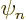with eigen­value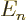, and that you are close to it, but no cigar. Then the wave func­tion can be writ­ten as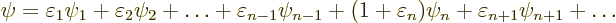whereis the one you want and the re­main­ing terms to­gether are the small er­ror in wave func­tion, writ­ten in terms of the eigen­func­tions. Their co­ef­fi­cients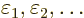are small.

The nor­mal­iza­tion con­di­tion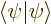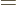1 is, us­ing or­tho­nor­mal­ity: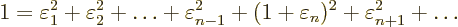The ex­pec­ta­tion en­ergy is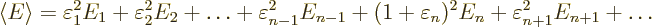or plug­ging in the nor­mal­iza­tion con­di­tion to elim­i­nate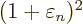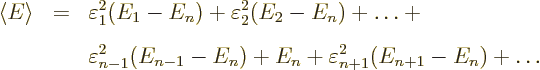As­sum­ing that the en­ergy eigen­val­ues are arranged in in­creas­ing or­der, the terms be­forein this sum are neg­a­tive and the ones be­hindpos­i­tive. Sois nei­ther a max­i­mum nor a min­i­mum; de­pend­ing on con­di­tions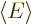can be greater or smaller than.

Now, if you make small changes in the wave func­tion, the val­ues ofwill slightly change, by small amounts that will be in­di­cated by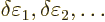, and you get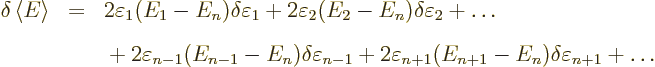This is zero when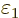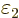0, so when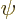is the ex­act eigen­func­tion. And it is nonzero as soon as any ofis nonzero; a change in that co­ef­fi­cient will pro­duce a nonzero change in ex­pec­ta­tion en­ergy. So the vari­a­tional con­di­tion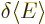0 is sat­is­fied at the ex­act eigen­func­tion, but not at any nearby dif­fer­ent wave func­tions.

The bot­tom line is that if you lo­cate the near­est wave func­tion for which0 for all ac­cept­able small changes in that wave func­tion, well, if you are in the vicin­ity of an en­ergy eigen­func­tion, you are go­ing to find that eigen­func­tion.

One fi­nal note. If you look at the ex­pres­sion above, it seems like none of the other eigen­func­tions are eigen­func­tions. For ex­am­ple, the ground state would be the case thatis one, and all the other co­ef­fi­cients zero. So a small change inwould seem to pro­duce a changein ex­pec­ta­tion en­ergy, and the ex­pec­ta­tion en­ergy is sup­posed to be con­stant at eigen­states.

The prob­lem is the nor­mal­iza­tion con­di­tion, whose dif­fer­en­tial form says that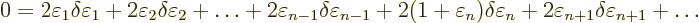At1 and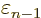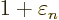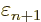0, this im­plies that the change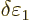must be zero. And that means that the change in ex­pec­ta­tion en­ergy is in fact zero. You see that you re­ally need to elim­i­natefrom the list of co­ef­fi­cients near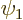, rather than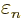as the analy­sis fordid, for the math­e­mat­ics not to blow up. A co­ef­fi­cient that is not al­lowed to change at a point in the vicin­ity of in­ter­est is a con­fus­ing co­ef­fi­cient to work with.